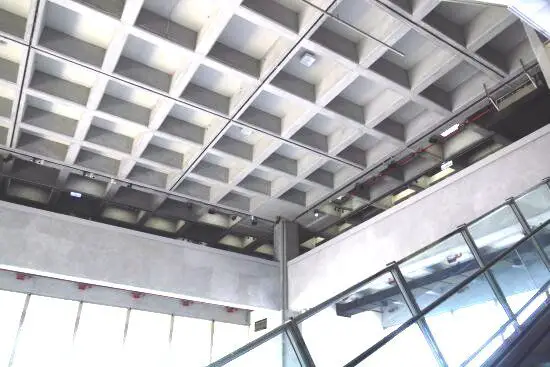# Example on Structural Design of Waffle SlabWaffle slabs can be described as the equivalent of 2-way solid slabs especially when the spans are large, and ribbed system is to be adopted. The design of waffle slabs is the same as that of ribbed slabs, with the difference being that waffle slabs have ribs spanning in both directions, and the coefficients used for analysing the slab is similar to those used for two-way restrained slab. Waffle slabs are supported on beams or columns, where the support zones are made to be uniformly thick.

Design Example
A church building has a square grid of 7.5m x 7.5m of waffle slab, and is to support an imposed load of 5 kN/m2, design an interior panel of the waffle slab, and a supporting beam. fck = 30 Mpa, fyk = 500 Mpa.

For details of waffle mould, see details of technical data sheet attached.

Solution
From basic span/effective depth ratio of 26, the trial depth of slab to be adopted = 7500/26 = 288 mm

From the table, let us select the following mould details;
Mould height = 225 mm
Top layer thickness = 75 mm
Total height of slab = 300 mm
Average rib width = 176 mm

Self weight of concrete = 5.2 kN/m2 (see technical data sheet)
Finishes = 1.2 kN/m2
Partition allowance = 1.5 kN/m2
Total permanent action = 7.9 kN/m2

At ultimate limit state = 1.35gk + 1.5qk = 1.35(7.9) + 1.5(5) = 18.165 kN/m2

Load per rib = 0.9 × 18.165 = 16.35 kN/m2
Analysis of the slab
Treating as all sides fixed:
k = Ly/Lx = 1.0

Span coefficient = +0.024
Support coefficients = -0.032

Design of the span
Designing the span as a T-beam;
MEd =  0.024 × 16.35 × 7.5= 22.07 kN.m

Effective depth (d) = h – Cnom – ϕ/2 – ϕlinks
Assuming ϕ12 mm bars will be employed for the main bars, and ϕ10 mm bars for the stirrups (links)
d = 300 – 25 – (12/2) -10 = 259 mm

k = MEd/(fckbd2) = (22.07 × 106)/(30 × 500 × 2592) = 0.0219
Since k < 0.167, no compression reinforcement required

z = d[0.5+ √(0.25 – 0.882k)]
k’ = 0.0219
z = d[0.5+ √((0.25 – 0.882(0.0219))] = 0.95d = 246.05 mm

Depth to neutral axis x = 2.5(d – z) = 2.5(259 – 246.05) = 32.375 mm < 1.25hf (93.75 mm)
Therefore, we design rib as a rectangular section

Area of tension reinforcement As1 = MEd / (0.87fyk z)
As1 = MEd / (0.87fyk z) = (22.07 × 106) / (0.87 × 500 × 0.95 × 259) = 206 mm2

Provide 2H12  Bot (ASprov = 226 mm2)

Check for deflection
ρ = As,req /bd = 206 / (900 × 259) = 0.0008837
ρ0 = reference reinforcement ratio = 10-3√(fck) = 10-3√(30) = 0.00547
Since  ρ ≤ ρ0;
L/d = k [11 + 1.5√(fck) ρ0/ρ + 3.2√(fck) (ρ0/ρ – 1)(3⁄2)]
k = 1.5 (continuous system)
L/d = 1.5 [11 + 1.5√(30) × (0.00547/0.0008837) + 3.2√(30) × [(0.00547 / 0.0008837) – 1](3⁄2)]
L/d = 1.5[11 + 50.855 + 207.227] = 269.082

βs = (500 Asprov)/(fyk Asreq) = (500 × 226) / (500 × 206) = 1.09

beff/bw = 900/176 = 5.11

Therefore multiply basic length/effective depth ratio by 0.8

Therefore limiting L/d = 1.09 × 0.8  × 269.082 = 234.639
Actual L/d = 7500/259 = 28.96

Since Actual L/d (28.96) < Limiting L/d (234.639), deflection is satisfactory.

Design of Supports;
MEd = 0.032 × 16.35 × 7.5= 29.43 kN.m

k = MEd/(fckbd2) = (29.43 × 106)/(30 × 176 × 2592) = 0.083
Since k < 0.167, no compression reinforcement required

z = d[0.5+ √(0.25 – 0.882k)]
k = 0.083
z = d[0.5+ √((0.25 – 0.882(0.083))] = 0.92d

Area of tension reinforcement As1 = MEd / (0.87fyk z)
As1 = MEd / (0.87fyk z) = (29.43 × 106) / (0.87 × 500 × 0.92 × 259) = 283 mm2
Provide 3H12 TOP (ASprov = 339 mm2)

Shear Design
Maximum shear force in the rib VEd = 0.33 × 16.35 × 7.5 = 40.466 kN/m

VRd,c = [CRd,c.k.(100ρ1 fck)(1/3) + k1cp]bw.d ≥ (Vmin + k1cp) bw.d

CRd,c = 0.18/γc = 0.18/1.5 = 0.12
k = 1 + √(200/d) = 1 + √(200/259) = 1.88 < 2.0, therefore, k = 1.88
Vmin = 0.035k(3/2) fck0.5
Vmin = 0.035 × (1.88)1.5 × 300.5 = 0.494 N/mm2
ρ1 = As/bd = 339/(176 × 259) = 0.00743 < 0.02; Therefore take 0.00743

VRd,c = [0.12 × 1.88 (100 × 0.00743 × 30 )(1/3)] × 176 × 259 = 28941.534 N = 228.94 kN

Since VRd,c (28.94 kN) < VEd (40.466 kN), shear reinforcement is required.
The compression capacity of the compression strut (VRd,max) assuming θ = 21.8° (cot θ = 2.5)

VRd,max = (bw.z.v1.fcd) / (cot⁡θ + tanθ)
V1 = 0.6(1 – fck/250) = 0.6(1 – 30/250) = 0.528
fcd = (αcc fck) / γc = (0.85 × 30) / 1.5 = 17 N/mm2
Let z = 0.9d

VRd,max = [(176 × 0.9 × 259 × 0.528 × 17) / (2.5 + 0.4)] × 10-3 = 126.981 kN

Since < VEd < VRd,c < VRd,max

Hence Asw / S = VEd / (0.87 Fyk z cot θ) = 40466 / (0.87 × 500 × 0.9 × 259 × 2.5 ) = 0.159

Minimum shear reinforcement;
Asw / S = ρw,min × bw × sinα (α = 90° for vertical links)
ρw,min = (0.08 × √(fck)) / fyk = (0.08 × √30) / 500 = 0.000876
Asw/Smin = 0.000876 × 176 × 1 = 0.154
Maximum spacing of shear links = 0.75d = 0.75 × 259 = 194.25 mm

Provide H8mm @ 175 mm c/c as shear links (Asw / S = 0.574)

Slab Topping
A142 BRC Mesh can be provided or H8 @ 250mm c/c

Watch out for design of supporting beams…

#### 1 COMMENT

1.relu59

Thank you for this information!

Ps.
1. For various reasons I cannot access the information in the link for: “technical data sheet attached.”
…. if you can send me this information on the email address: relu59@yahoo.com.

2. I have to solve urgently a floor with dimensions 7.80 m x 24.20 m leaning on a structure of load-bearing masonry with the thickness of walls of 0.45 m. Are you willing to give me some advice or even technical solutions ?